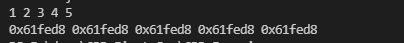Initialize an integer array of 5 elements. Then, print values of all elements along with their addresses using pointers.

#include <iostream>
#include <array>
using namespace std;

int main()
{
array<int, 5> arr = {1, 2, 3, 4, 5};
array<int, 5> *arr2 = &arr;
for (int i:arr)
{
cout <<i << " ";
}
cout << endl;
for (int i:*arr2)
{
cout <<arr2 << " ";
}
return 0;
}cpp programming exercise with solution